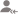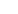true

Take Class 11 Tuition from the Best Tutors

•Affordable fees
•1-1 or Group class
•Flexible Timings
•Verified Tutors

Search in# Learn Equilibrium with Free Lessons & Tips

Post a Lesson

CaC03(s) → CaO(s) + C02(g),∆fH° = -635.1 kJ mol--1,∆fH° = -393.5 kJ mol--1,∆fH° = -1206.9 kJ mol--1... read more
CaC03(s) → CaO(s) + C02(g),∆fH° [CaO(s)] = -635.1 kJ mol–1,∆fH° [COz(g)] = -393.5 kJ mol–1,∆fH° [CaC03(s)] = -1206.9 kJ mol–1 Predict the effect of temperature on the equilibrium constant of the above reaction. read less

A reaction between ammonia and boron trifluoride is given below: :NH3 + BF3 →H3N : BF3. Identify the... read more
A reaction between ammonia and boron trifluoride is given below: :NH3 + BF3 →H3N : BF3. Identify the acid and base in this reaction. Which theory explains it? What is the hybridization of B and N in the reactants? read less

Calculate the volume of water required to dissolve 0.1 g lead (II) chloride to get a saturated solution.... read more
Calculate the volume of water required to dissolve 0.1 g lead (II) chloride to get a saturated solution. (Ksp of PbCl2 = 3.2 x 10-8, atomic mass of Pb = 207 u). read less

Take Class 11 Tuition from the Best Tutors

•Affordable fees
•Flexible Timings
•Choose between 1-1 and Group class
•Verified Tutors

The solubility product of Al(OH)3 is 2.7 x 10-11. Calculate its solubility in g--L and also find out... read more
The solubility product of Al(OH)3 is 2.7 x 10-11. Calculate its solubility in g–L and also find out pH of this solution. (Atomic mass of A1 = 27 u). read less

Calculate the pH of a solution formed by mixing equal volumes of two solutions A and B of a strong acid... read more
Calculate the pH of a solution formed by mixing equal volumes of two solutions A and B of a strong acid having pH = 6 and pH = 4 respectively. read less

pH of 0.08 mol dm–3HOC1 solution is 2.85. Calculate its ionization constant.

Take Class 11 Tuition from the Best Tutors

•Affordable fees
•Flexible Timings
•Choose between 1-1 and Group class
•Verified Tutors

A sparingly soluble salt gets precipitated only when the product of concentration of its ions in the... read more
A sparingly soluble salt gets precipitated only when the product of concentration of its ions in the solution (Qsp) becomes greater than its solubility product. If the solubility of BaS04 in water is 8 x 10-4 mol dm-3, calculate its solubility in 0.01 mol dm-3 of H2S04. read less

pH of a solution of a strong acid is 5.0. What will be the pH of the solution obtained after diluting... read more
pH of a solution of a strong acid is 5.0. What will be the pH of the solution obtained after diluting the given solution a 100 times? read less

On the basis of the equation pH = -log , the pH of 10-8 mol dm-3 solution of HC1 should be 8. However,... read more
On the basis of the equation pH = -log [H+], the pH of 10-8 mol dm-3 solution of HC1 should be 8. However, it is observed to be less than 7.0. Explain the reason. read less

Take Class 11 Tuition from the Best Tutors

•Affordable fees
•Flexible Timings
•Choose between 1-1 and Group class
•Verified Tutors

The value of Kc for the reaction 2HI(g) ⇌H2(g) + I2(g) is 1 x 10-4. At a given time, the composition... read more
The value of Kc for the reaction 2HI(g) ⇌H2(g) + I2(g) is 1 x 10-4. At a given time, the composition of reaction mixture is [HI] = 2 x 10-5 mol, [H2] = 1 x 10-5 mol and [I2] = 1 x 10-5 mol. In which direction will the reaction proceed? read less

UrbanPro.com helps you to connect with the best Class 11 Tuition in India. Post Your Requirement today and get connected.

Overview

Questions 129

Total Shares58 Followers

## Class 11 Tuition in:

x

X

### Looking for Class 11 Tuition Classes?

The best tutors for Class 11 Tuition Classes are on UrbanPro

• Select the best Tutor
• Book & Attend a Free Demo
• Pay and start Learning### Take Class 11 Tuition with the Best Tutors

The best Tutors for Class 11 Tuition Classes are on UrbanPro